# Pandas Dataframe Align function

Pandas Align basically helps to align the two dataframes have the same row and/or column configuration and as per their documentation it Align two objects on their axes with the specified join method for each axis Index. We use align when we would like to synchronize a dataframe with another dataframe or a dataframe with a Series or in other words we want to map one dataframe into another dataframe or series using different join methods like outer, inner, left and right.

#### Original Tables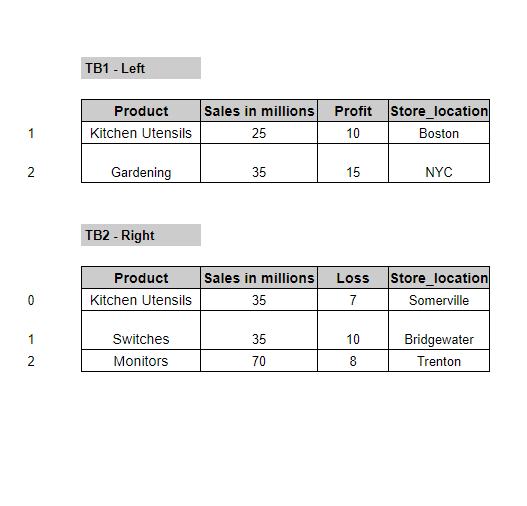#### Align with Left Join on Columns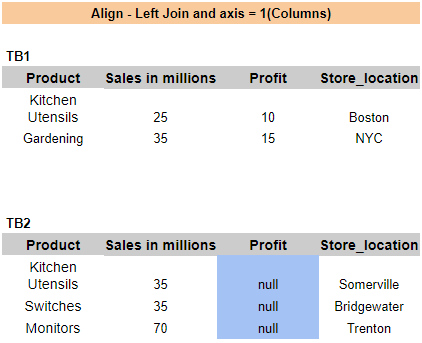#### Align with Inner Join on Columns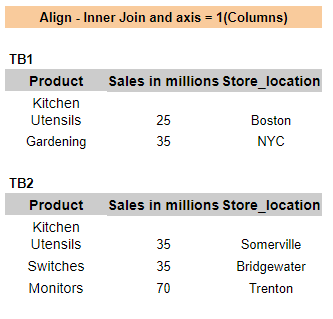#### Align with Right Join on Columns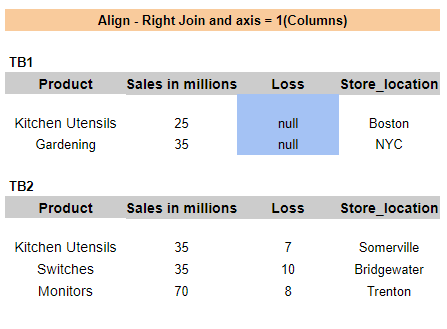#### Align with Left Join on Index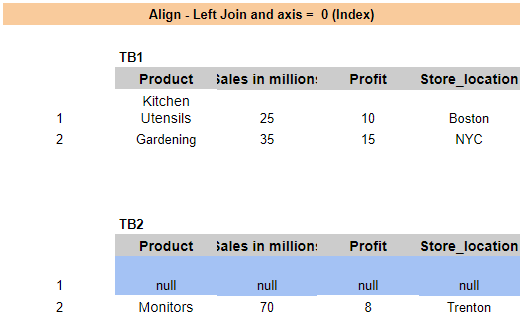Lets understand this with the help of some examples:

First create two dataframes with first dataframe having columns Product, Sales, Profit and Store Location and two rows at index 1,2 and Second dataframe with columns Product, Sales, Loss and Store Location and three rows at index 2,3 and 4

``````df1 = pd.DataFrame([['Kitchen Utensils',25,10,'Boston'], ['Gardening',35,15,'NYC']], columns=['Product', 'Sales in millions', 'Profit', 'Store_location'], index=[1,2])
df2 = pd.DataFrame([['Kitchen Utensils',35,7,'Somerville'], ['Switches',35,10,'Bridgewater'], ['Monitors',70,8,'Trenton']], columns=['Product', 'Sales in millions', 'Loss', 'Store_location'], index=[2,3,4])
``````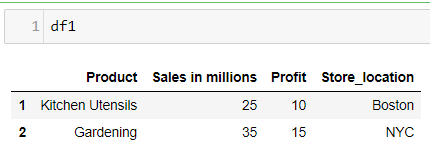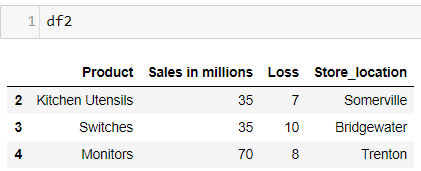## Align - Right Join

We want to align the first dataframe(left) with the second dataframe(right). So here I would be using the right Join which means it will sync the first dataframe with the second one and all columns of right(second dataframe) will be present in the first dataframe. Column Loss is added to the first dataframe(left) and the valus are NaN and column Profit is deleted from that dataframe because Profit column is not available in the right Dataframe(second df)

``````a1, a2 = df1.align(df2, join='right', axis=1)
print(a1)
print(a2)
``````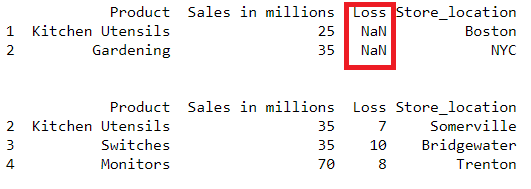We will see later in this post on how to fill the NaN values in these aligned columns

## Align - Left Join

Another example, where we want to align the second dataframe(left) with the first dataframe(right). So here I would be using the left Join which means it will sync the second dataframe with the first one and all columns of left(first dataframe) will be present in the second dataframe. Column Profit is added to the first dataframe(left) and the valus are NaN and column Profit is deleted from that dataframe because Profit column is not available in the left Dataframe(first df)

We will see later in this post on how to fill the NaN values in these aligned columns

``````a1, a2 = df1.align(df2, join='left', axis=1)
print(a1)
print(a2)
``````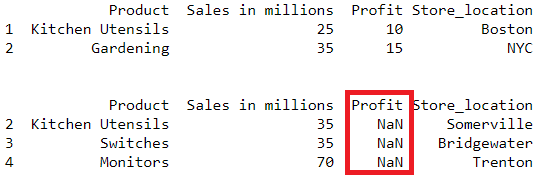## Change Axis

Change axis to Zero if you want to align on Index. Since we have a left join here so df2 index should match with df1 index. df1 have index 1 and 2 therefore df2 will have these two indexes after alignment and all the values for df2 index 1 will be NaN and index 2 value will be as it is.

``````a1, a2 = df1.align(df2, join='left', axis=0)
print(a1)
print(a2)
``````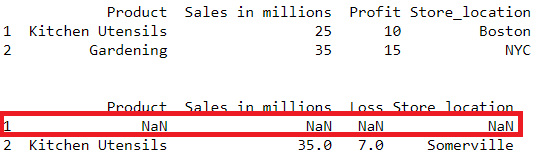## Align - Inner Join

Lets see what happens if we set the join as inner here.It will try to match the rows and columns which are same in both the left and right dataframes. Row at Index 2 and all three columns are same between both the dataframes and that is restored and rest all other indexes and columns like profit and loss is dropped

``````a1, a2 = df1.align(df2, join='inner', axis=None)
print(a1)
print(a2)
``````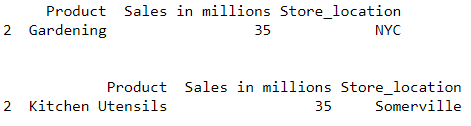Now lets see how to fill the NaN values and what are the methods that is provided with this function to backfill or forwardfill or set a default values for these NaN values.

Look at our first example where we did a left join and a null column profit is created in dataframe 2. Now if you want to fill these NaN values then there are other parameters available in this function which can be really useful here.

## Replace Left Join NaN with Default Values

All the values in column Profit will be filled with the default value

``````a1, a2 = df1.align(df2, join='left', axis=1, fill_value = 90 )
print(a1)
print(a2)
``````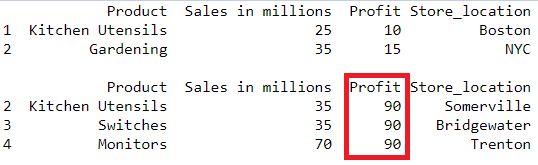## Fill the NaN values using any of the methods like backfill or forward fill.

### bfill - backfill Example

if you want to fill the NaN with the values from next row then use this method with axis = 0

``````a1, a2 = df1.align(df2, join='left', axis=0, method = 'bfill', fill_axis = 0)
print(a1)
print(a2)
``````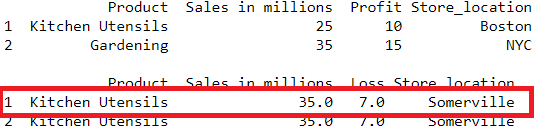### ffill - forward fill Example

if you want to fill the NaN with the values from next row then use this method with axis = 1

``````a1, a2 = df1.align(df2, join='left', axis=1, method = 'ffill', fill_axis = 1 )
print(a1)
print(a2)
``````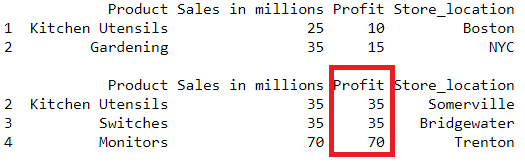Tags:

Categories:

Updated: